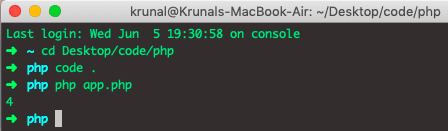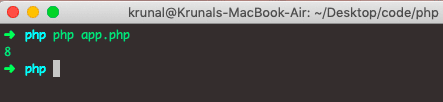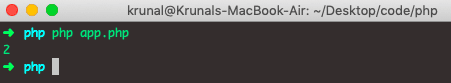# PHP Array Count Example | PHP count() Function TutorialPHP Array Count Example | PHP count() Function Tutorial is today’s topic. PHP count() function returns the number of items in the array. PHP array count() method is used to count the items of an array or the properties of an object.

For objects, if you have installed SPL, then you can hook into count() by implementing the interface Countable. The interface has only one method, Countable::count(), which returns a return value for the count() function.

## PHP Array Count Example

PHP count() function count all elements in an array or something in an object. See the following syntax.

``count(array,mode);``

The array parameter is required, and it returns the number of elements in the array.

The mode parameter is Optional. Specifies the mode. Possible values:

1. 0 – Default. Does not count all elements of multidimensional arrays
2. 1 – Counts the array recursively (counts all the elements of multidimensional arrays)

See the following code example of PHP count() method.

``````<?php

// app.php

\$brands = ['Louis Vuitton', 'Gucci', 'Prada', 'Dior'];
echo count(\$brands);``````

See the below output.In the above code, we have four items in the array. So, it will return 4 in the output.

The count() function may return 0 for the variable which is not set, but it may also return 0 for the variable that has been initialized with the empty array.

The isset() function should be used to test whether the variable is set or not.

## Recursive count() example

See the following code.

``````<?php

// app.php

\$food = array('fruits' => array('orange', 'banana', 'apple'),
'veggie' => array('carrot', 'collard', 'pea'));

echo count(\$food, COUNT_RECURSIVE);
``````

Now run the app.php file and see the output.In the above code, we have passed the COUNT_RECURSIVE parameter that is why it counts every item in the array as well as sub-arrays that is why the total 8 items and it returns 8.

If you do not pass the COUNT_RECURSIVE, then it will return 2 items.

``````<?php

// app.php

\$food = array('fruits' => array('orange', 'banana', 'apple'),
'veggie' => array('carrot', 'collard', 'pea'));

echo count(\$food);
``````

See the output.If you want to run through the large arrays then don’t use the count() function in the loops, its an over the head in performance,  copy the count() value into a variable and use that value in loops for better performance.

See the following code example.

``````// Bad approach

for(\$i=0;\$i<count(\$arr);\$i++)
{
// calculations
}

// Good approach

\$arr_length = count(\$arr);
for(\$i=0;\$i<\$arr_length;\$i++)
{
// calculations
}``````

The following function of one line to find a number of items that are not arrays, recursively.

``````function count_elt(\$array, &\$count=0){
foreach(\$array as \$v) if(is_array(\$v)) count_elt(\$v,\$count); else ++\$count;
return \$count;
}``````

When it comes to multidimensional arrays, and you do not want all levels of the array tree. The following function is beneficial.

``````<?php

// \$limit is set to the number of recursions

function count_recursive (\$array, \$limit) {
\$count = 0;
foreach (\$array as \$id => \$_array) {
if (is_array (\$_array) && \$limit > 0) {
\$count += count_recursive (\$_array, \$limit - 1);
} else {
\$count += 1;
}
}
return \$count;
}
?>``````

Finally, PHP Array Count Example | PHP count() Function Tutorial is over.